•5星
29KB m0_52957036 2020-05-09 20:12:07
•5星
11.8MB guoruibin123 2021-05-21 15:45:32
• MATLAB程序分享MATLAB实现线性拟合和相关系数源程序代码-MATLAB实现线性拟合和相关系数 源程序代码.rar matlab

2KB weixin_39840914 2019-08-13 11:21:12
• 75.14MB weixin_38624914 2021-06-20 04:23:34
• 296B drjiachen 2019-09-06 16:08:00
• matlab拟合非线性和线性代码 曲线拟合

3.64MB qq_30072889 2017-11-26 13:04:09
• 线性回归置信区间：计算并绘制线性拟合可能所在的置信区间。-matlab开发 matlab

2KB weixin_38530995 2021-05-30 15:44:10
• Matlab线性拟合和非线性拟合 Matlab 线性拟合 非线性拟合

线性拟合 已知如下图像的x,y坐标，x = [1.0, 1.5, 2.0, 2.5, 3.0]，y = [0.9, 1.7, 2.2, 2.6, 3.0]，如何用一条直线去拟合下列散点？ 代码： x = [1.0, 1.5, 2.0, 2.5, 3.0]'; y = [0.9, 1.7, 2.2, 2.6, 3.0]'...

线性拟合

已知如下图像的x,y坐标，x = [1.0, 1.5, 2.0, 2.5, 3.0]，y = [0.9, 1.7, 2.2, 2.6, 3.0]，如何用一条直线去拟合下列散点？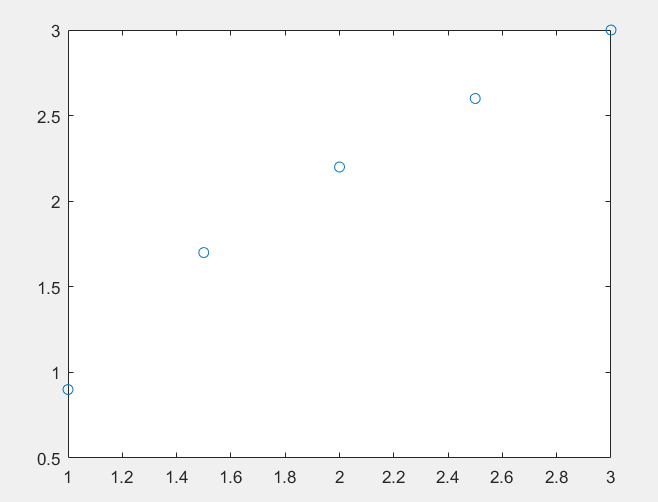代码：

x = [1.0, 1.5, 2.0, 2.5, 3.0]';
y = [0.9, 1.7, 2.2, 2.6, 3.0]';
a = polyfit(x,y,1)  % a会返回两个值，[斜率，x=0时y的值]
xi = 1:0.1:3;
yi = polyval(a,xi);
plot(x,y,'o',xi,yi);

拟合后的图像如下：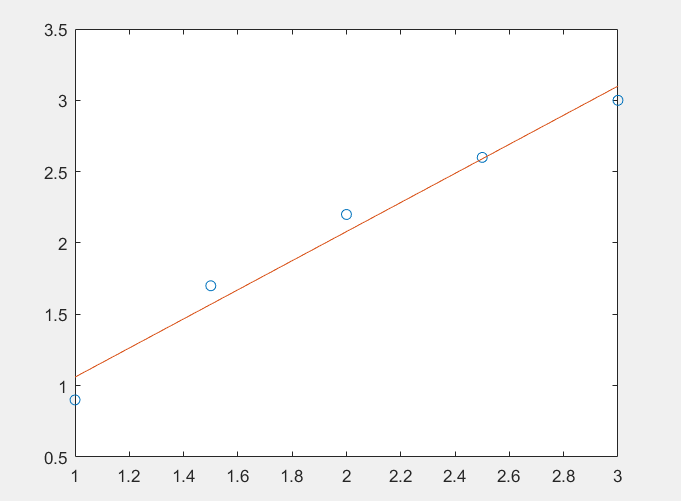非线性拟合

普通非线性拟合：已知如下图像的x,y坐标，x = [1.0, 1.5, 2.0, 2.5, 3.0]，y = [0.9, 1.7, 2.2, 2.6, 3.0]，如何用一条曲线a*x+b*sin(x)+c去拟合下列散点？代码：

x = [1.0, 1.5, 2.0, 2.5, 3.0]';
y = [0.9, 1.7, 2.2, 2.6, 3.0]';
p = fittype('a*x+b*sin(x)+c');
f = fit(x,y,p)   % f会返回a,b,c的值
plot(f,x,y);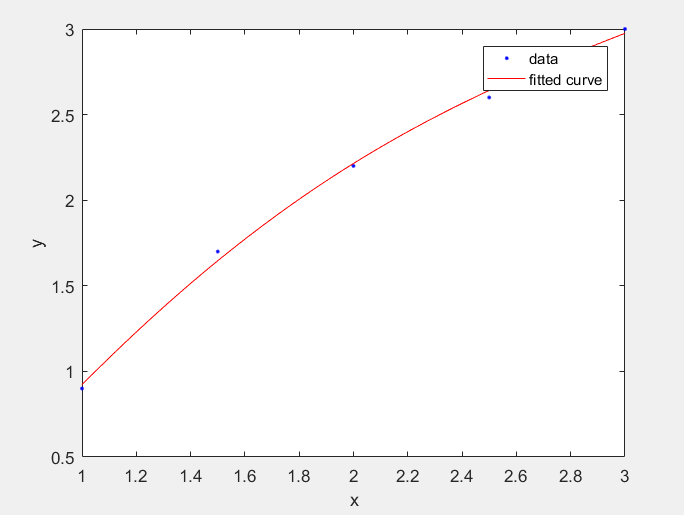指数非线性拟合：如何拟合1790-1900年美国人口指数增长模型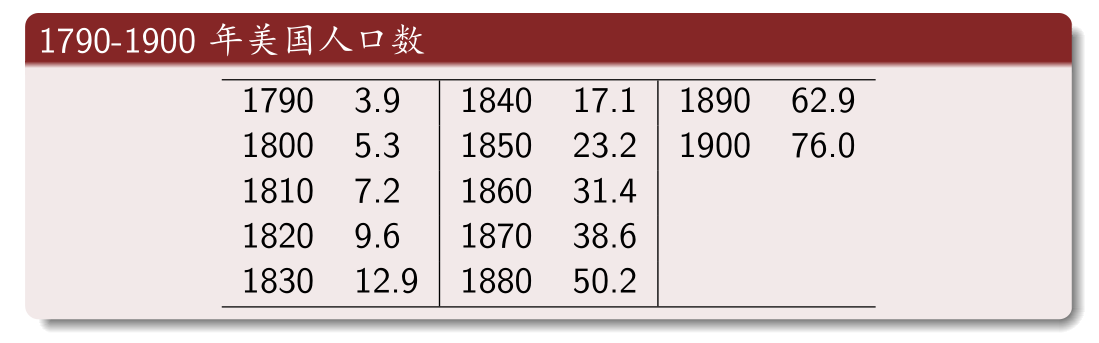思路：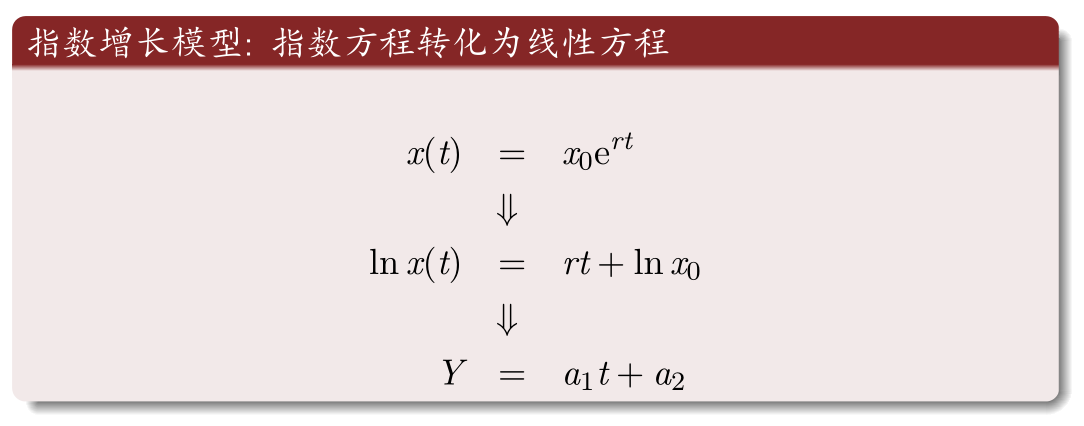实现代码：

t = 1790:10:1900;
p = [3.9 5.3 7.2 9.6 ...
12.9 17.1 23.2 31.4 ...
38.6 50.2 62.9 76.0];
Y = log(p); % Y = log(p) 返回数组p中每个元素的自然对数ln(x)
X = t;
a = polyfit(X,Y,1)
x0 = exp(a(2)); r = a(1);
ti = 1790:1900;
pti= x0*exp(r*ti);
plot(t,p,'o',ti,pti,'m')
xlabel('Year')
ylabel('Population')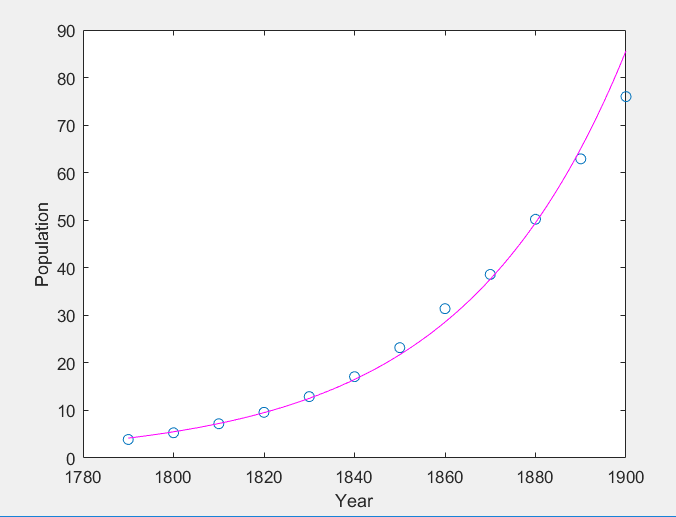对数形式的非线性拟合：

%% 对数形式非线性回归
x = [1.5, 4.5, 7.5,10.5,13.5,16.5,19.5,22.5,25.5];
y = [7.0,4.8,3.6,3.1,2.7,2.5,2.4,2.3,2.2];
plot(x, y, '*', 'linewidth', 1) % 这里的linewidth指的是散点大小
m1 = @(b,x) b(1) + b(2)*log(x);
nonlinfit1 = fitnlm(x,y,m1,[0.01;0.01])
b = nonlinfit1.Coefficients.Estimate;
Y1 = b(1,1) + b(2,1)*log(x);
hold on
plot(x, Y1, '--k', 'linewidth',2)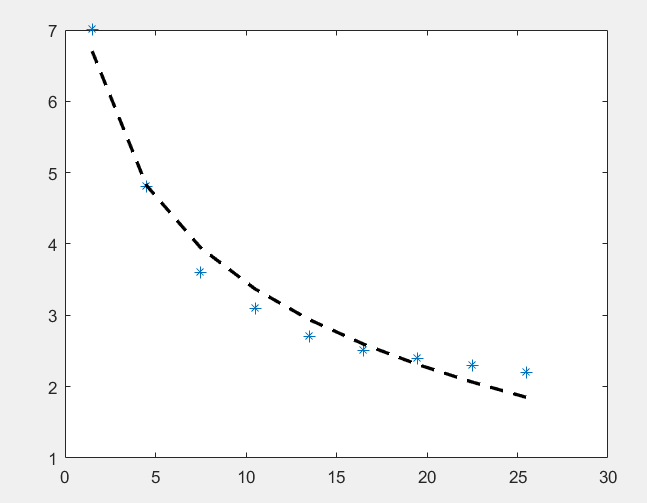展开全文qq_41149269 2019-01-23 09:25:36
• 413B sky_goodstudy 2017-05-16 09:16:56
• 一、单一变量的曲线逼近Matlab有一个功能强大的曲线拟合工具箱 cftool ，使用方便，能实现多种类型的线性、非线性曲线拟合。下面结合我使用的 Matlab R2007b 来简单介绍如何使用这个工具箱。假设我们要拟合的函数...

一、 单一变量的曲线逼近

Matlab有一个功能强大的曲线拟合工具箱 cftool ，使用方便，能实现多种类型的线性、非线性曲线拟合。下面结合我使用的 Matlab R2007b 来简单介绍如何使用这个工具箱。

假设我们要拟合的函数形式是 y=A*x*x + B*x, 且A>0,B>0 。

1、在命令行输入数据：

》x=[110.3323 148.7328 178.064 202.8258033 224.7105 244.5711 262.908 280.0447 296.204 311.5475]；

》y=[5 10 15 20 25 30 35 40 45 50]；

2、启动曲线拟合工具箱

》cftool

3、进入曲线拟合工具箱界面“Curve Fitting tool”

(1)点击“Data”按钮，弹出“Data”窗口；

(2)利用X data和Y data的下拉菜单读入数据x,y，可修改数据集名“Data set name”，然后点击“Create data set”按钮，退出“Data”窗口，返回工具箱界面，这时会自动画出数据集的曲线图；

(3)点击“Fitting”按钮，弹出“Fitting”窗口；

(4)点击“New fit”按钮，可修改拟合项目名称“Fit name”，通过“Data set”下拉菜单选择数据集，然后通过下拉菜单“Type of fit”选择拟合曲线的类型，工具箱提供的拟合类型有：

Custom Equations：用户自定义的函数类型

Exponential：指数逼近，有2种类型， a*exp(b*x) 、 a*exp(b*x) + c*exp(d*x)

Fourier：傅立叶逼近，有7种类型，基础型是 a0 + a1*cos(x*w) + b1*sin(x*w)

Gaussian：高斯逼近，有8种类型，基础型是 a1*exp(-((x-b1)/c1)^2)

Interpolant：插值逼近，有4种类型，linear、nearest neighbor、cubic spline、shape-preserving

Polynomial：多形式逼近，有9种类型，linear ~、quadratic ~、cubic ~、4-9th degree ~

Power：幂逼近，有2种类型，a*x^b 、a*x^b + c

Rational：有理数逼近，分子、分母共有的类型是linear ~、quadratic ~、cubic ~、4-5th degree ~；此外，分子还包括constant型

Smoothing Spline：平滑逼近(翻译的不大恰当，不好意思)

Sum of Sin Functions：正弦曲线逼近，有8种类型，基础型是 a1*sin(b1*x + c1)

Weibull：只有一种，a*b*x^(b-1)*exp(-a*x^b)

选择好所需的拟合曲线类型及其子类型，并进行相关设置：

——如果是非自定义的类型，根据实际需要点击“Fit options”按钮，设置拟合算法、修改待估计参数的上下限等参数；

——如果选Custom Equations，点击“New”按钮，弹出自定义函数等式窗口，有“Linear Equations线性等式”和“General Equations构造等式”两种标签。

在本例中选Custom Equations，点击“New”按钮，选择“General Equations”标签，输入函数类型y=a*x*x + b*x，设置参数a、b的上下限，然后点击OK。

(5)类型设置完成后，点击“Apply”按钮，就可以在Results框中得到拟合结果，如下例：

general model:

f(x) = a*x*x+b*x

Coefficients (with 95% confidence bounds):

a = 0.009194 (0.009019, 0.00937)

b = 1.78e-011 (fixed at bound)

Goodness of fit:

SSE: 6.146

R-square: 0.997

RMSE: 0.8263

同时，也会在工具箱窗口中显示拟合曲线。

这样，就完成一次曲线拟合啦，十分方便快捷。当然，如果你觉得拟合效果不好，还可以在“Fitting”窗口点击“New fit”按钮，按照步骤(4)~(5)进行一次新的拟合。

不过，需要注意的是，cftool 工具箱只能进行单个变量的曲线拟合，即待拟合的公式中，变量只能有一个。对于混合型的曲线，例如 y = a*x + b/x ，工具箱的拟合效果并不好。

展开全文weixin_33657090 2021-04-26 11:42:18
• 596B u011889953 2013-08-30 15:35:36
• 2KB xiaoshuoyimei 2014-09-15 20:08:29
• 展开全部matlab中线性拟合可以使来用多项自式拟合函数polyfit()实现bai，du其调用格式为P=polyfit(X,Y,N)其中zhi，X，Y是需要拟合的数据，N是拟dao合的多项式的最高次数，P是拟合的多项式的系数。实例演示如下：1、...

展开全部

matlab中线性拟合可以使来用多项自式拟合函数polyfit()实现bai，du其调用格式为P = polyfit(X,Y,N)

其中zhi，X，Y是需要拟合的数据，N是拟dao合的多项式的最高次数，P是拟合的多项式的系数。

实例演示如下：

1、示例数据

x=[0.1,0.2,0.3,0.4,0.5,0.6,0.7,0.8,0.9,1.0,1.1,1.2]

y=[-8,-236,-415,-562,-701,-860,-961,-1082,-1188,-1304,-1405,-1534]

拟合方程：y=a+bx

2、matlab代码>> x=[0.1,0.2,0.3,0.4,0.5,0.6,0.7,0.8,0.9,1.0,1.1,1.2];

>> y=[-8,-236,-415,-562,-701,-860,-961,-1082,-1188,-1304,-1405,-1534];

>> p = polyfit(x,y,1)

>> plot(x,y,'o',x,polyval(p,x))

3、结果及说明p =

1.0e+003 *

-1.3253    0.0068

表示拟合的线性公式为 ： y = -1325.3x+6.8展开全文weixin_39960319 2020-12-24 03:59:08
• 5.04MB weixin_38685961 2021-06-07 23:11:13
• 802B qq_36851515 2018-09-18 09:33:25
• 30KB weixin_38514872 2021-05-22 21:42:17
• 95.67MB weixin_38677044 2021-05-22 21:41:08
• Matlab 线性拟合 polyfit 结果不准/偏差很大 matlab polyfit 线性拟合

由于我的数据来源于实验，太大没法放在这里，直接放代码和图展示问题，记此笔记方便后续翻阅 [T30H_Lin, F30H_Lin] = SelectFitData(0.65,2.9,F30H, T30H); [p,S,mu] = polyfit(T30H_Lin,F30H_Lin,1); % 函数调用...

polyfit 结果不准

由于我的数据来源于实验，太大没法放在这里，直接放代码和图展示问题，记此笔记方便后续翻阅

[T30H_Lin, F30H_Lin] = SelectFitData(0.65,2.9,F30H, T30H);
[p,S,mu] = polyfit(T30H_Lin,F30H_Lin,1);  % 函数调用方式与下面有区别
T30H_Lin_Fit = T30H_Lin(1):0.1:20;
F30H_Lin_Fit = polyval(p,T30H_Lin_Fit);

plot(T30H_Lin_Fit, F30H_Lin_Fit)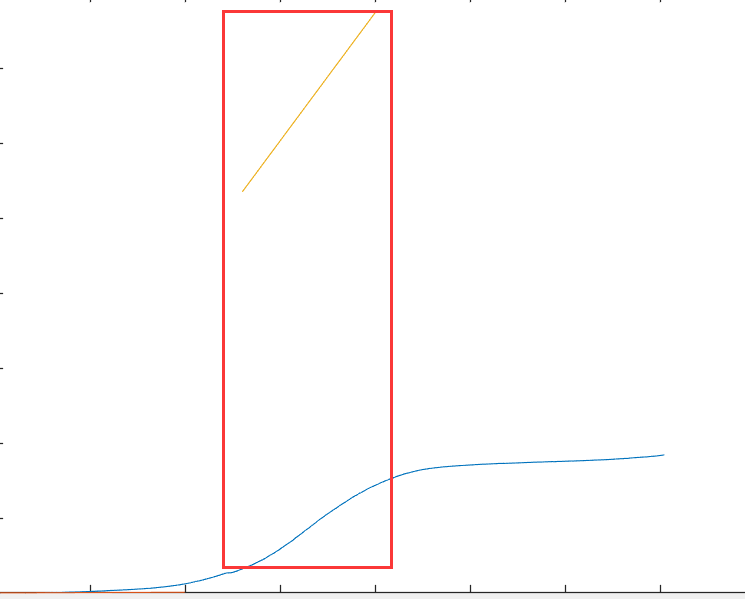[T30H_Lin, F30H_Lin] = SelectFitData(0.65,2.9,F30H, T30H);
p = polyfit(T30H_Lin,F30H_Lin,1);
T30H_Lin_Fit = T30H_Lin(1):0.1:20;
F30H_Lin_Fit = polyval(p,T30H_Lin_Fit);

plot(T30H_Lin_Fit, F30H_Lin_Fit)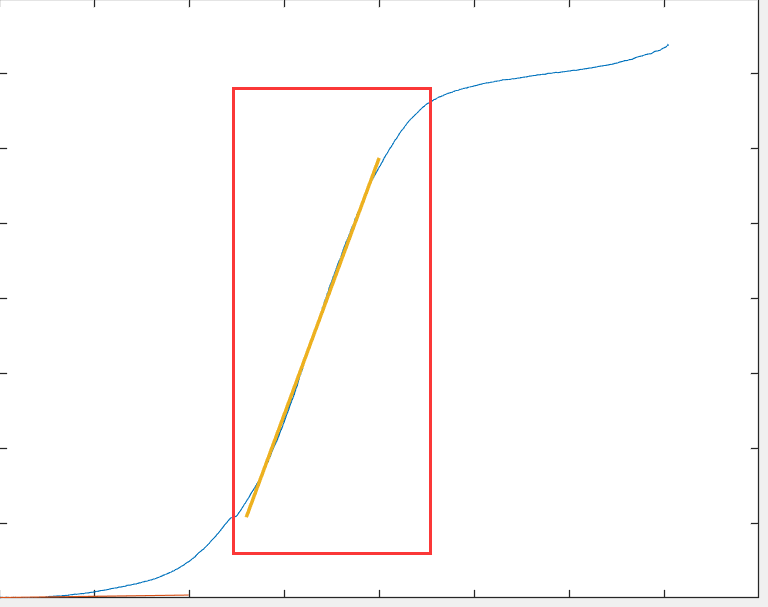结论

p = polyfit(T30H_Lin,F30H_Lin,1);
[p,S,mu] = polyfit(T30H_Lin,F30H_Lin,1);

这两种调用方式结果不一样，不应该认为返回值返回值越多越精确或者说返回值越多的肯定包含少的

展开全文jh1513 2020-03-19 16:58:11
• matlab非线性拟合程序代码 matlab4星
12KB zjws_6688 2008-06-10 17:44:28
• MATLAB求解非线性最小二乘法拟合问题 源程序代码 matlab

2KB ddpiccolo 2019-03-18 23:54:02
• 2KB weixin_38745434 2021-05-21 06:00:23
• 多元线性回归matlab代码 测绘工程

1KB qq_43225085 2018-10-19 17:48:44
• weixin_42395669 2021-04-20 10:48:20
• 2.68MB weixin_38647039 2021-05-26 21:02:29
• 23KB weixin_38606300 2021-05-22 21:42:22
• 174.96MB weixin_38609913 2021-05-22 21:42:14
• weixin_42521276 2021-04-20 04:13:34
• matlab代码---非线性拟合 matlab

qq_43362405 2020-04-16 11:54:38
• qq_45458915 2020-04-20 16:59:39
• Matlab实现一元线性拟合 matlab 数学建模

weixin_45614076 2021-07-24 16:51:45
• 3KB qq_31880107 2019-08-31 15:51:57
• 利用matlab实现非线性拟合（三维、高维、参数方程） matlab 非线性拟合 线性拟合

weixin_42943114 2021-05-14 19:41:29...

matlab线性拟合代码matlab 订阅